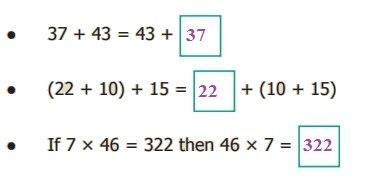Home | | Maths 6th Std | Solving unknowns through examples

# Solving unknowns through examples

Introduction To Algebra : Solving unknowns through examples

Solving unknowns through examples

Let us fill up the empty boxesThestands for an unknown number.

To make the equations meaningful, we shall write 5 in the first box, we shall write 7 in the second box and we shall write 6 in the third box.

Try these

Find the unknown.Example 2.1

Suppose that there are some eggs in a tray. If 6 eggs are taken out from it and still 10 eggs are remaining, how many eggs are there in the tray?According to the given statement,This can be written as, x – 6 gives 10, where ‘x’ denotes the unknown number.

Now, we will find out for what value of ‘x’, x − 6 gives 10.Hence, the unknown number (variable) ‘x’ takes the value 16.

Try this

Find the suitable value of ‘m’, to get a sum of 9?Example 2.2

Athiyan and Mugilan are brothers. Athiyan is ‘p’ years old and Mugilan is elder to Athiyan by 6 years. Write an algebraic statement for this and find the age of Mugilan if Athiyan is 20 years old.

Age of Athiyan             = ‘p’ years

Age of Mugilan             = ‘p + 6’ years (algebraic statement)

If p = 20, then Mugilan’s age is = 20 + 6

= 26 years.

Tags : Introduction to Algebra | Term 1 Chapter 2 | 6th Maths , 6th Maths : Term 1 Unit 2 : Introduction To Algebra
Study Material, Lecturing Notes, Assignment, Reference, Wiki description explanation, brief detail
6th Maths : Term 1 Unit 2 : Introduction To Algebra : Solving unknowns through examples | Introduction to Algebra | Term 1 Chapter 2 | 6th Maths